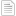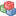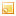# A modified approach based on ranking fuzzy numbers for fuzzy integer programming with equality constraints

.

Díaz-Madroñero M, Mula J, Jiménez M. 2012. A modified approach based on ranking fuzzy numbers for fuzzy integer programming with equality constraints. XVI Congreso de Ingeniería de Organización , pag. 375-382. Vigo.

###Resumen

This paper proposes a method for solving fuzzy integer programming problems where all the cost coefficients of the objective function and the right hand side terms of equality constraints are, in general, fuzzy numbers. We formulate a modified fuzzy ranking method to rank the fuzzy objective values and to deal with the equality relation on constraints under integrity conditions. We build a fuzzy subset in the integer decision space whose membership function represents the balance between the feasibility degree of constraints and the satisfaction degree of the goal. Finally, to illustrate our proposal, we solve a numerical example of a transport planning problem.

###Congreso

(cio2012)XVI Congreso de Ingeniería de Organización

###Area

EN-03. Production & Operations Management

###Palabras Clave

• Fuzzy integer programming
• ranking fuzzy numbers
• equality constraints
• transport planning problem
• uncertainty